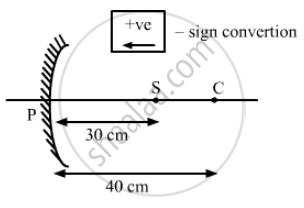Department of Pre-University Education, KarnatakaPUC Karnataka Science Class 12
Advertisement Remove all ads

# A Concave Mirror Having a Radius of Curvature 40 Cm is Placed in Front of an Illuminated Point Source at a Distance of 30 Cm from It. Find the Location of the Image. - Physics

Sum

A concave mirror having a radius of curvature 40 cm is placed in front of an illuminated point source at a distance of 30 cm from it. Find the location of the image.

Advertisement Remove all ads

#### Solution

Using sign conventions, given,
Distance of object from mirror, u = − 30 cm,
Radius of curvature of concave mirror R = − 40 cmUsing the mirror equation,

$\frac{1}{v} + \frac{1}{u} = \frac{2}{R}$

$\Rightarrow \frac{1}{v} = \frac{2}{R} - \frac{1}{u}$

$\Rightarrow \frac{1}{v} = \frac{2}{- 40} - \frac{1}{- 30} = \frac{1}{- 20} + \frac{1}{30}$

$\Rightarrow \frac{1}{v} = \frac{- 30 + 20}{30 \times 20} = \frac{- 10}{30 \times 20}$

$\Rightarrow \frac{1}{v} = - \frac{1}{60}$

or, v = − 60 cm
Hence, the required image will be located at a distance of 60 cm in front of the concave mirror.

Is there an error in this question or solution?
Advertisement Remove all ads

#### APPEARS IN

HC Verma Class 11, 12 Concepts of Physics 1
Chapter 18 Geometrical Optics
Q 1 | Page 412
Advertisement Remove all ads
Advertisement Remove all ads
Share
Notifications

View all notifications

Forgot password?